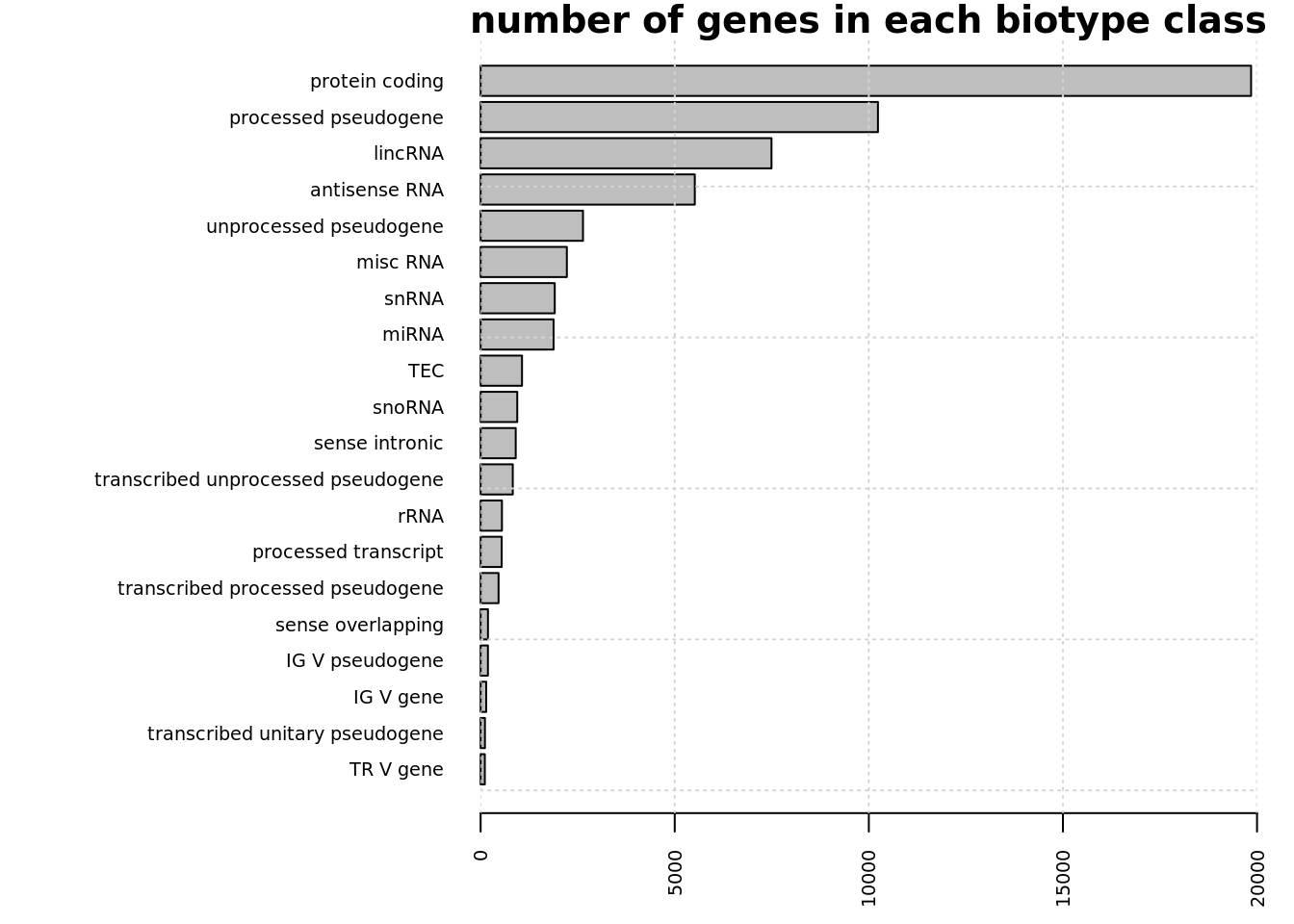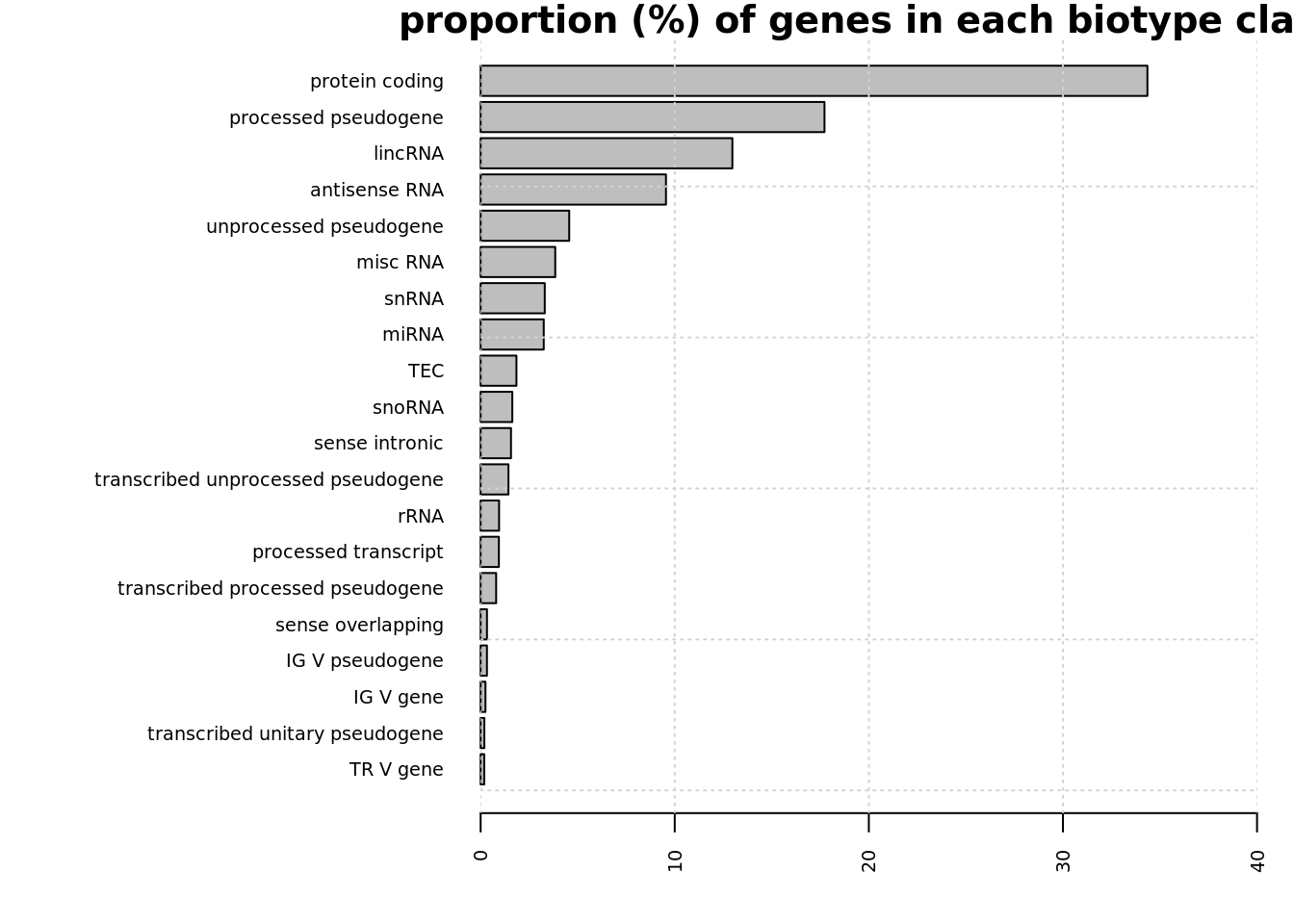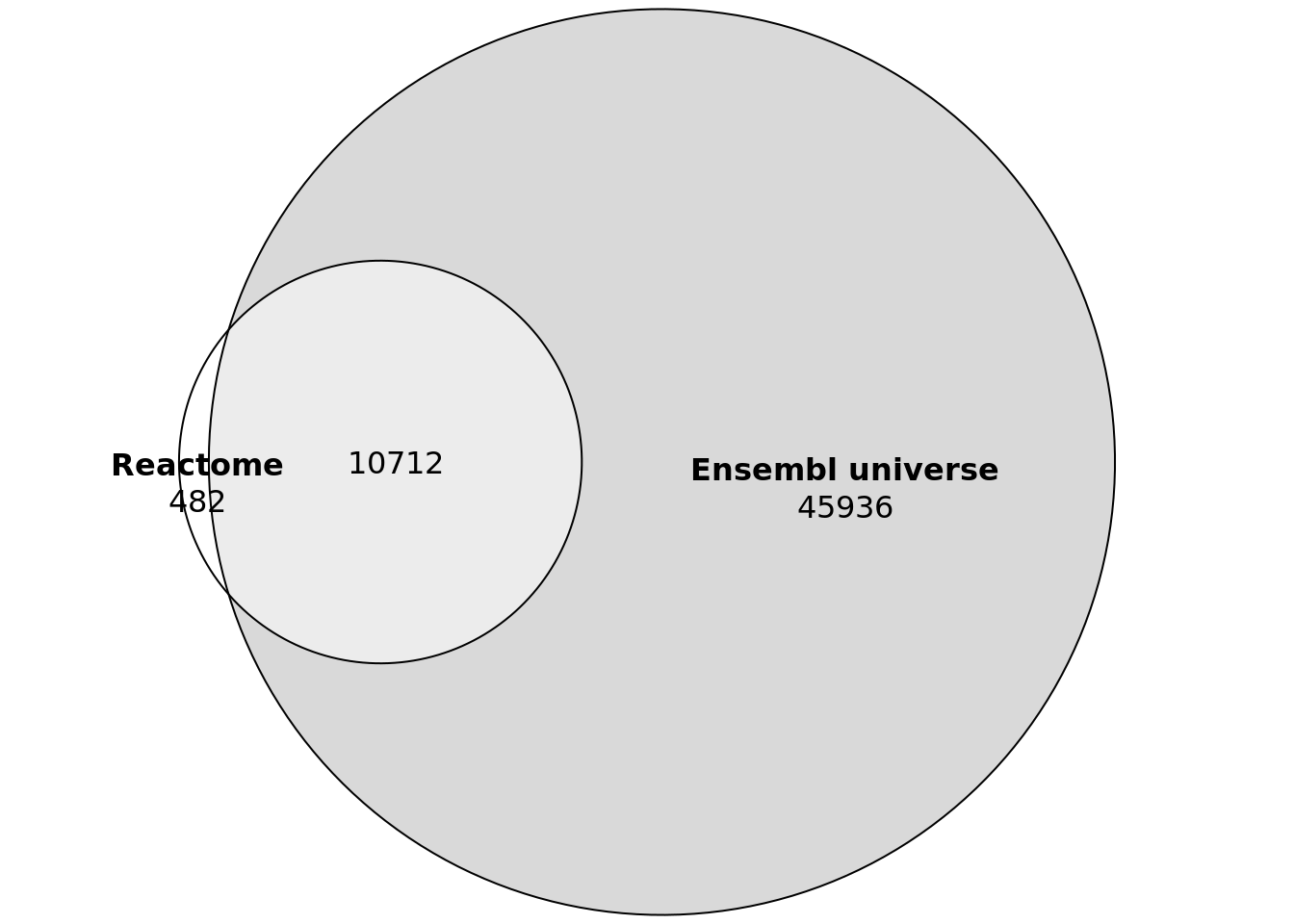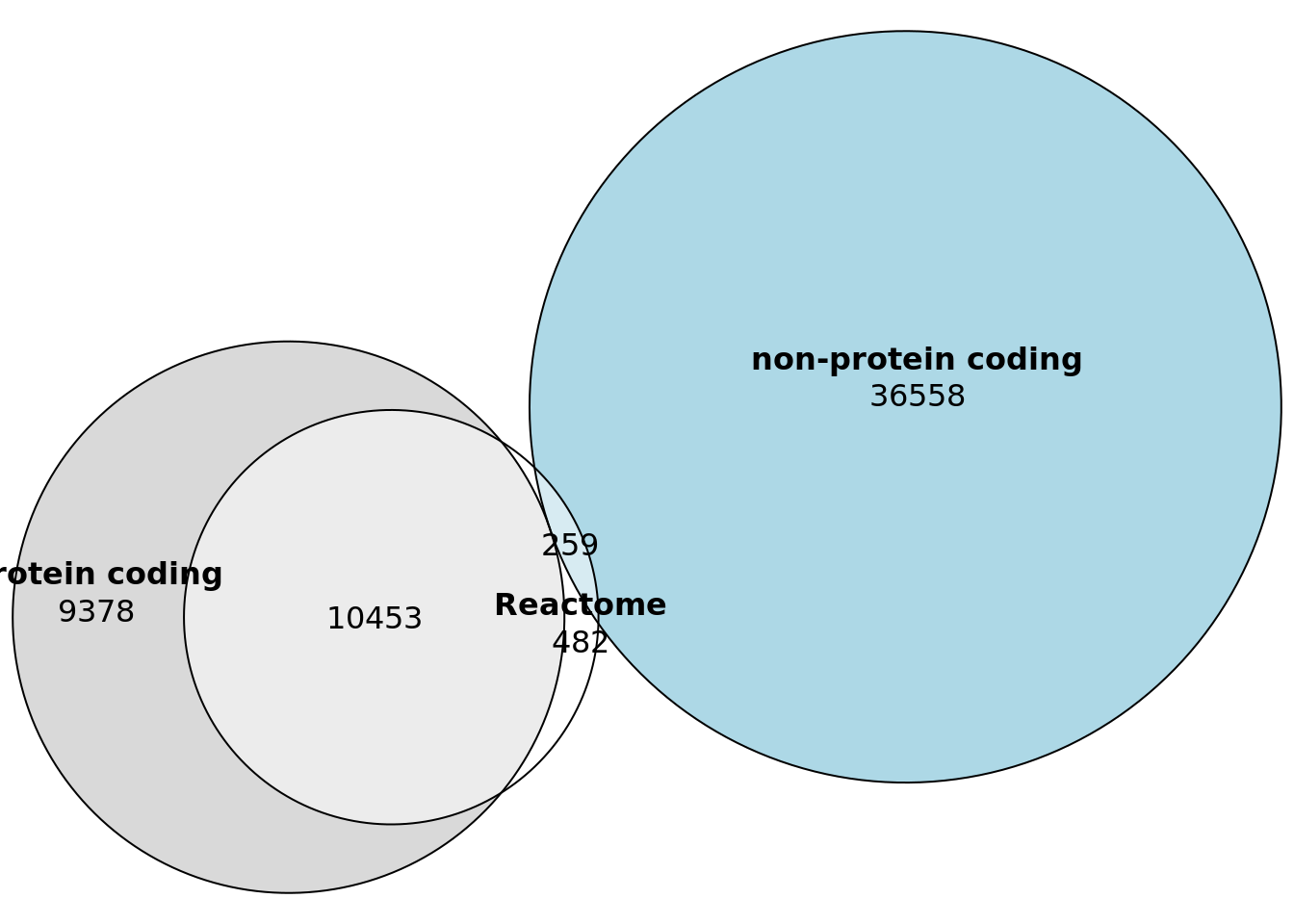## Background

In this analysis we will be characterising the new gene sets.

## Libraries

``````suppressPackageStartupMessages({
library("getDEE2")
library("mitch")
library("triwise")
library("dplyr")
library("gplots")
library("reshape2")
library("network")
library("eulerr")
})``````

## Reference gene list

This is the background - all genes in the "universe" according to Ensembl version 90, which is the same annotation set used by DEE2. You can see that there are a large number of non-protein coding genes.

``````# get universe of gene names and biotypes
if (! file.exists("hs.gtf.gz") ) {
}

g <- g[grep("gene",g\$V3),9]
universe <- sapply(strsplit(g," "),"[[",6)
universe <- gsub(";","",universe)

biotypes <- sapply(strsplit(g," "),"[[",10)
biotypes <- gsub("_"," ",biotypes)
biotypes <- gsub(";","",biotypes)

biotypes_df <- data.frame(universe,biotypes)

universe <- unique(universe)

mytable <- table(biotypes)
mytable <- mytable[order(mytable)]
mytable <- mytable[which(mytable>100)]

par(mar=c(3,13,1,1)); barplot(mytable,horiz=TRUE,las=2,cex.names = 0.6,cex.axis = 0.6,
main="number of genes in each biotype class",xlim = c(0,20000)) ;grid()````````````mytable <- mytable/sum(mytable)*100

par(mar=c(3,13,1,1)); barplot(mytable,horiz=TRUE,las=2,cex.names = 0.6,cex.axis = 0.6,
main="proportion (%) of genes in each biotype class" ,xlim=c(0,40)) ;grid()````````````# Take note how many transcripts are protein coding versus non-protein coding
paste("protein coding:",length(which(biotypes=="protein coding")))``````
``##  "protein coding: 19847"``
``paste("non-protein coding:",length(which(biotypes!="protein coding")))``
``##  "non-protein coding: 38455"``

## Curated gene sets

### Reactome

Here I download the current Reactome gene set library. I would like to see how many Ensembl genes have some sort of annotated function. Breaking it down into protein coding and non-protein coding, we can see that non-protein coding genes are severely underrepresented in Reactome. Reactome has 2400 sets and a total of 11193 genes as of 29/Sep/2020.

``````#o
#unzip("ReactomePathways.gmt.zip")
reactome <- gmt_import("ReactomePathways.gmt")
reactome_genes <- unique(unname(unlist(reactome)))
length(reactome)``````
``##  2408``
``length(reactome_genes)``
``##  11194``
``````v1 <- list("Reactome"=reactome_genes, "Ensembl universe"=universe)
library("eulerr")
plot(euler(v1),quantities = TRUE)````````length(reactome_genes)/length(universe)*100``
``##  19.76063``
``````prot <- biotypes_df[which(biotypes_df\$biotypes=="protein coding"),1]
prot <- unique(prot)
nprot <- biotypes_df[which(biotypes_df\$biotypes!="protein coding"),1]
nprot <- unique(nprot)
intersect(prot,nprot)``````
``````##   "RGS5"        "TMEM247"     "CYB561D2"    "CDR1"        "KBTBD11-OT1"
##   "MAL2"        "SPATA13"     "SFTA3"       "GOLGA8M"     "ELFN2"``````
``````nprot <-   setdiff(nprot,prot)
v1 <- list("Reactome"=reactome_genes, "protein coding"=prot, "non-protein coding"=nprot)
plot(euler(v1),quantities = TRUE)``````### Gene Ontology

Now let's do the same with GO sets downloaded from Ensembl biomart. GO has 12264 sets and 8412 genes as of 26/Sep/2020.

``go <- read.table("biomart_2020-09-26.txt.gz",sep="\t", fill = TRUE)``
``````## Warning in scan(file = file, what = what, sep = sep, quote = quote, dec = dec, :
## EOF within quoted string``````
``````colnames(go) <- go[1,]
go <- go[2:nrow(go),]
go <- go[which(go\$`GO term accession`!=""),]
go_genes <- unique(go\$`Gene name`)
length(unique(go\$`GO term accession`))``````
``##  12264``
``length(go_genes)``
``##  8412``
``````v1 <- list("GO"=go_genes, "protein coding"=prot, "non-protein coding"=nprot)
plot(euler(v1),quantities = TRUE)``````